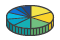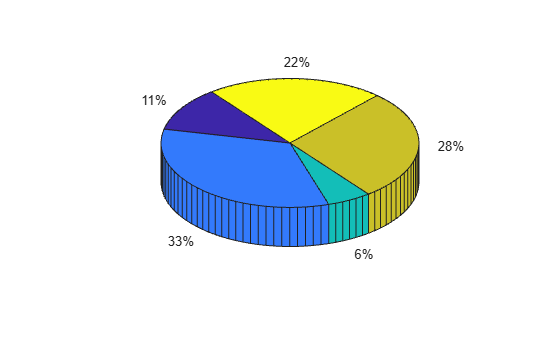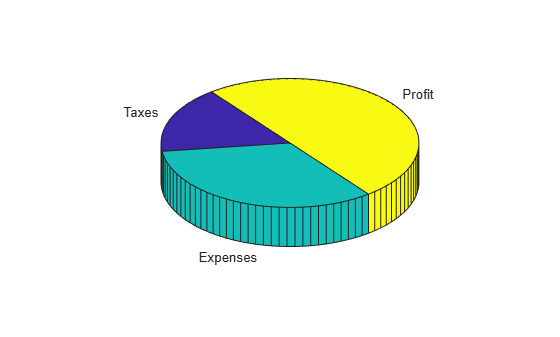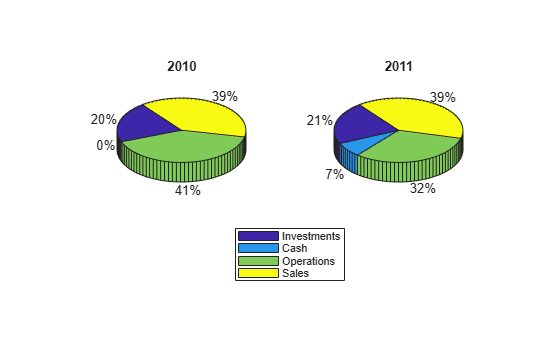pie3

•Syntax

pie3(X)
pie3(X,explode)
pie3(...,labels)
pie3(axes_handle,...)
h = pie3(...)

Description

pie3(X) draws a three-dimensional pie chart using the data in X. Each element in X is represented as a slice in the pie chart.

• If sum(X) ≤ 1, then the values in X directly specify the area of the pie slices. pie3 draws only a partial pie if sum(X) < 1.

• If the sum of the elements in X is greater than one, then pie3 normalizes the values by X/sum(X) to determine the area of each slice of the pie.

pie3(X,explode) specifies whether to offset a slice from the center of the pie chart. X(i,j) is offset from the center of the pie chart if explode(i,j) is nonzero. explode must be the same size as X.

pie3(...,labels) specifies text labels for the slices. The number of labels must equal the number of elements in X.

pie3(axes_handle,...) plots into the axes with the handle axes_handle instead of into the current axes (gca).

h = pie3(...) returns a vector of handles to patch, surface, and text graphics objects.

Examples

collapse all

Create a 3-D pie chart of vector x.

x = [1,3,0.5,2.5,2];
figure
pie3(x)To offset the second pie slice, set the corresponding explode element to 1.

explode = [0,1,0,0,0];
figure
pie3(x,explode)Create a 3-D pie chart and specify the text labels.

x = 1:3;
labels = {'Taxes','Expenses','Profit'};

figure
pie3(x,labels)Create vectors y2010 and y2011, that contain financial data for two years. Then create a cell array containing the labels for the values.

y2010 = [50 0 100 95];
y2011 = [65 22 97 120];
labels = {'Investments','Cash','Operations','Sales'};

Create a 2-by-1 tiled chart layout, and display a pie chart and legend for y2010 data in the first tile. Then display a pie chart and legend for the y2011 data in the second tile.

t = tiledlayout (2,1);
ax1 = nexttile;
pie3(ax1,y2010)
title('2010')
legend(labels)

ax2 = nexttile;
pie3(ax2,y2011)
title('2011')
legend(labels)Compatibility Considerations

expand all

Behavior changed in R2019b